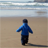Announcements
Applications are open for the 2024 Qlik Luminary Program. Apply by December 15 here.
cancel
Showing results for
Did you mean:Creator

## YTD Calculation

Hi All,

I am new to qlik sense.

I have a requirement to calculate YTD.

I have two Fields

The Link_Fiscal_Period has values from 2018001/2018002/2018003 and so on....

The LINK_FISCAL_PERIOD_DATE has values from 'Apr-2017'/May-2017/Jun-2017 and so on..

I wrote an expression to calculate YTD as below but expression is not resulting any values.

Could you please check and let me know is there any issue with expression or do the expression requires any tweaks to get the desired values.

Note : LINK_FISCAL_PERIOD date format is CCYY0MM

The Mapping of these fields are as below:

if(FISCAL_YEAR_PERIOD = '2018001', 'Apr-2017',

if(FISCAL_YEAR_PERIOD = '2018002', 'May-2017',

if(FISCAL_YEAR_PERIOD = '2018003', 'Jun-2017',

if(FISCAL_YEAR_PERIOD = '2018004', 'Jul-2017',

if(FISCAL_YEAR_PERIOD = '2018005', 'Aug-2017',

if(FISCAL_YEAR_PERIOD = '2018006', 'Sep-2017',

if(FISCAL_YEAR_PERIOD = '2018007', 'Oct-2017',

if(FISCAL_YEAR_PERIOD = '2018008', 'Nov-2017',

if(FISCAL_YEAR_PERIOD = '2018009', 'Dec-2017',

if(FISCAL_YEAR_PERIOD = '2018010', 'Jan-2018',

if(FISCAL_YEAR_PERIOD = '2018011', 'Feb-2018',

if(FISCAL_YEAR_PERIOD = '2018012', 'Mar-2018',

if(FISCAL_YEAR_PERIOD = '2019001', 'Apr-2018',

if(FISCAL_YEAR_PERIOD = '2019002', 'May-2018',

if(FISCAL_YEAR_PERIOD = '2019003', 'Jun-2018',

if(FISCAL_YEAR_PERIOD = '2019004', 'Jul-2018',

if(FISCAL_YEAR_PERIOD = '2019005', 'Aug-2018',

if(FISCAL_YEAR_PERIOD = '2019006', 'Sep-2018',

if(FISCAL_YEAR_PERIOD = '2019007', 'Oct-2018',

if(FISCAL_YEAR_PERIOD = '2019008', 'Nov-2018',

if(FISCAL_YEAR_PERIOD = '2019009', 'Dec-2018',

if(FISCAL_YEAR_PERIOD = '2019010', 'Jan-2019',

if(FISCAL_YEAR_PERIOD = '2019011', 'Feb-2019',

if(FISCAL_YEAR_PERIOD = '2019012', 'Mar-2019',

Thanks,

S k

10 RepliesMaster II

First change your date format to either MM/DDYYYY or DD/MM/YYYY to make it easier

2nd create Year, Month and Quarters from your date field. or MonthYear field

To get YTD dynamically use

Sum({ <Month = {"<=\$(=num(Month))"},>}YourFieldHere)

Month field above is your Jan,Feb,Mar etc...

So whatever month you select, it will show the data for selected month and previous months in selected year which would be YTD.

if July-2018 is selected, it will show from Jan-2018 to July-2018.

I'd suggest explain with a small XL file data with expected output. You can create Year,Month, Quarters etc within your fact if you have a date field.Tags
Community Browser